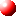English \$B!C(B Home \$B!C(B Our Group | NTT CS Labs.\$B>7BT9V1i(B\$B!!(B
• "AI\$B\$,3+\$/L\$Mh!A(BAI\$B3hMQ\$N>-MhE8K>!A(B"(\$BBg:eI\9)6H6(2q(B AI\$B8&5f2q(B, \$B1w!'Bg:eI\9)6H6(2q(B), 2019\$BG/(B3\$B7n(B5\$BF|(B.

• "\$B?M9)CNG=\$,\$b\$?\$i\$9L\$Mh
• "\$B?M9)CNG=\$r;Y\$(\$k5!3#3X=,5;=Q\$N8=>u\$H>-MhE8K>(B"(\$B%G!<%?!&%\$%s%F%j%8%'%s%9650i8&5fItLg%;%_%J!<(B, \$B1w!'OB2N;3Bg3X(B), 2018\$BG/(B11\$B7n(B27\$BF|(B.

• "\$B?M9)CNG=5;=Q\$N?7E83+(B"(\$BEE5\$;03X2q4X@>;YIt(B \$B=Z0w\$*\$h\$S3X@8\$N\$?\$a\$N9V1i2q(B, \$B1w!'6a5&Bg3X(B), 2018\$BG/(B11\$B7n(B12\$BF|(B.

• "\$B?M9)CNG=\$r3hMQ\$7\$?L\$Mh\$BBh(B61\$B2s7zC[;N2qA49qBg2q!V\$5\$\$\$?\$^Bg2q!W(B, \$B1w!'Bg5\%=%K%C%/%7%F%#(B), 2018\$BG/(B10\$B7n(B26\$BF|(B.

• "\$BBh(B4\$B
• "Simulation-based Machine Learning"(The 2nd International Workshop for symbolic-Neural Learning (SNL2018)  \$B1w!'L>8E209q:]2q5D>l(B), 2018\$BG/(B7\$B7n(B6\$BF|(B.

• "\$B?M9)CNG=\$N<+A32J3X!"2018\$BG/EY?M9)CNG=3X2qA49qBg2q(B(\$BBh(B32\$B2s(B)\$B%A%e!<%H%j%"%k9V1i(B, \$B1w!'>k;3%[%F%k
• "\$BJ#;(!&9bEY\$J
• "AI\$B5;=Q\$,@Z\$jBs\$/L\$Mh\$NKI:R5;=Q\$HE8K>(B"(\$BBh(B17\$B2s(B \$B9qEZ%;%\$%U%F%#%M%C%H%7%s%]%8%&%`(B, \$B1w!'%Q%7%U%#%32#IM(B), 2018\$BG/(B2\$B7n(B8\$BF|(B.

• "\$B%9!<%Q!<%3%s%T%e!<%?!<\$H?M9)CNG=5;=Q\$NM;9g\$K\$h\$k%7%_%e%l!<%7%g%s2J3X(B"(\$B6e=#Bg3X(B \$B?7%9!<%Q!<%3%s%T%e!<%?!
• "\$B?M9)CNG=5;=Q\$K\$h\$k\$,\$s8&5f\$N?7E83+(B"(\$B%7%s%]%8%&%`!V(BSociety5.0\$B;~Be\$N%X%k%9%1%"\$N:_\$jJ}!W(B, \$B1w!'El5~Bg3X(B \$B0KF#
• "\$BKI:RJ,Ln\$K\$*\$1\$k?M9)CNG=\$N3hMQ!!!AM}8&(B \$B3W?7CNG=E}9g8&5f%;%s%?!<(B \$BKI:R2J3X%A!<%`\$G\$NR2p!A(B"(\$B9q:]9bEy8&5f=j(B \$B%(%8%=%s\$N2q(B, \$B1w!'9q:]9bEy8&5f=j(B), 2017\$BG/(B12\$B7n(B26\$BF|(B.

• "\$B?M9)CNG=\$N4pAC5;=Q\$H\$=\$N1~MQ!!!AM}8&(BAIP\$B\$G\$N8&5f3+H/\$N\$4>R2p!A(B"(\$B;00f6H:]8&5f=j(B AI\$B5;=QD4::0Q0w2q9V1i2q(B, \$B1w!';00f6H:]8&5f=j(B), 2017\$BG/(B12\$B7n(B18\$BF|(B.

• "IoT\$B;~Be\$G\$N?M9)CNG=5;=Q\$H\$=\$N
• "AI\$B\$N:G?7F08~\$H:#8e\$N2DG=@-(B"(\$BBg:eI\9)6H6(2q(B \$B9)>l7P1D8&5f2q(B, \$B1w!'Bg:eI\9)6H6(2q(B), 2017\$BG/(B11\$B7n(B21\$BF|(B.

• "\$B?M9)CNG=;~Be\$r8#0z\$9\$k5!3#3X=,5;=Q(B"(\$BEE5\$;03X2q4X@>;YIt!V=Z0w\$*\$h\$S3X@80w\$N\$?\$a\$N9V1i2q(B, \$B1w!'4X@>Bg3X(B), 2017\$BG/(B11\$B7n(B16\$BF|(B.

• "\$B?M9)CNG=\$N?7E83+!!!A4D6-CNG=\$N\$BJ?@.(B29\$BG/EY(B \$BEZLZ8&5f=j9V1i2q(B, \$B1w!'0l669VF2(B), 2017\$BG/(B10\$B7n(B19\$BF|(B.

• "\$BM}2=3X8&5f=j!!3W?7CNG=E}9g8&5f%;%s%?!<\$N\$4>R2p(B"(\$BF|2C?M9)CNG=6(NO%U%)!<%i%`(B, \$B1w!':_F|%+%J%@Bg;H4[(B), 2017\$BG/(B10\$B7n(B4\$BF|(B.

• "AI\$B5;=Q\$,@8\$_\$@\$9L\$Mh\$BD+F|CO5e2q5D(B, \$B1w!'Dk9q%[%F%kEl5~(B), 2017\$BG/(B10\$B7n(B3\$BF|(B.

• "IoT\$B;~Be\$K\$*\$1\$k?M9)CNG=5;=Q\$N?7E83+(B"(\$BF`NI8);:6H?66=%;%s%?!<(B \$BAO@_(B100\$B<~G/5-G05;=Q%U%)!<%i%`(B, \$B1w!'F`NI8);:6H?66=%;%s%?!<(B), 2017\$BG/(B9\$B7n(B4\$BF|(B.

• "\$B5!3#3X=,\$N?7E83+(B \$B!A%7%_%e%l!<%7%g%s2J3X!A(B"(\$B5~!_%G!<%?%5%\$%(%s%9%7%s%]%8%&%`(B2017[\$B%G!<%?(Bmeets\$B%7%_%e%l!<%7%g%s(B], \$B1w!'4]%S%k%[!<%k(B), 2017\$BG/(B8\$B7n(B25\$BF|(B.

• "IoT\$B;~Be\$N5!3#3X=,5;=Q(B \$B!A4D6-CNG=\$NTohoku Forum for Creativity Symposium \$B%G!<%?\$H%\$%s%F%j%8%'%s%9(B, \$B1w!'ElKLBg3XJRJ?%-%c%s%Q%9(B \$BCN\$N4[(B), 2017\$BG/(B8\$B7n(B24\$BF|(B.

• "\$BJ#;(B?MM\$J%G!<%?\$+\$i\$N5!3#3X=,5;=Q(B \$B!A(BAI\$B;~Be\$N5!3#3X=,5;=Q\$N?7E83+!A(B"(\$B;0I)EE5!-j(B \$BDL?.5;=QIt2q(B \$B2hA|!&2;@
• "\$B?M9)CNG=8&5f\$N:GA0@~(B"(\$B%S%C%0%G!<%?%"%/%F%#%Y!<%7%g%s8&5f%;%s%?!<(B\$B!!%-%C%/%*%U%7%s%]%8%&%`(B, \$B1w!'?73cBg3XG>8&5f=j(B), 2017\$BG/(B7\$B7n(B21\$BF|(B.

• "\$B4D6-CNG=\$N
• "\$B%G!<%?\$K8l\$i\$;\$k2J3X!!!A(BIoT\$B;~Be\$+\$i\$N5!3#3X=,5;=Q(B"(JR\$BEl3\$(B \$BBh#3#02s5;=Q3+H/It%;%_%J!<(B,  \$B1w!'(BJR\$BEl3\$>.KR8&5f;\@_(B), 2017\$BG/(B5\$B7n(B29\$BF|(B.

• "\$B<+A32J3X!"\$B%7%s%]%8%&%`!V%G!<%?%5%\$%(%s%9\$N8=>u\$HL\$Mh(B\$B!W(B, \$B1w!'?@8MBg3X=P8w:4;05-G0O;9CBf9VF2(B), 2017\$BG/(B5\$B7n(B15\$BF|(B.

• "\$B3W?7E*?M9)CNG=\$N
• "\$BM}8&(BAIP\$B%;%s%?!<\$K\$*\$1\$kKI:R!&8::R8&5f\$N\$BET;T:R32%W%m%8%'%/%H(B \$B:G=*@.2LJs9p2q(B, \$B1w!'El5~Bg3X(B \$B0BED9VF2(B), 2017\$BG/(B3\$B7n(B14\$BF|(B.

• "\$B5!3#3X=,8&5f\$N?7E83+!!!]3FDEIM2017, \$B1w!'9b;3%0%j!<%s%[%F%k(B), 2017\$BG/(B3\$B7n(B6\$BF|(B.

• "\$B5!3#3X=,5;=Q\$K\$h\$k?M9)CNG=\$N?7E83+(B"(\$B%^%j%s%(%s%8%K%"%j%s%03X2q(B\$BFCJL4p6b9V1i2q(B, \$B1w!':{@n5-G02q4[(B), 2017\$BG/(B3\$B7n(B2\$BF|(B.

• "Recent novel applications based on advanced Machine Learning technologies"(MEET THE DATA \$B-7(B, \$B1w!'(BMaison De La Chimie), 2017\$BG/(B2\$B7n(B23\$BF|(B.

• "\$B5!3#3X=,5;=Q\$N;:6HE*3hMQ(B"(\$B:G?7?M9)CNG=(B(AI)\$B%7%s%]%8%&%`(B, \$B1w!'Bg:e2J3X5;=Q%;%s%?!<(B), 2017\$BG/(B2\$B7n(B10\$BF|(B.

• "\$B?M9)CNG=\$BEE;R>pJsDL?.3X2q!V%3%_%e%K%1!<%7%g%s%/%*%j%F%#4pAC9V:B%o!<%/%7%g%C%W!W(B, \$B1w!'Bg:eBg3X(B \$BCfG7Eg%;%s%?!<(B), 2017\$BG/(B1\$B7n(B21\$BF|(B.

• "\$B<+A32J3X\$BF|K\5!3#3X2q4X@>;YItFCJL%U%)!<%i%`(B, \$B1w!'Bg:e2J3X5;=Q%;%s%?!<(B), 2016\$BG/(B10\$B7n(B24\$BF|(B.

• "Recent Advancement in Machine Learning Research"(\$BEE;R>pJsDL?.3X2q(B \$B%R%e!<%^%s%3%_%e%K%1!<%7%g%s%0%k!<%W(B \$B>pJs\$NG'CN\$H9TF08&5f2q(B\$B%o!<%/%7%g%C%W(B, \$B1w!'5*=#FnIt%m%\$%d%k%[%F%k(B), 2016\$BG/(B10\$B7n(B6\$BF|(B.

• "\$BM}2=3X8&5f=j!!3W?7CNG=E}9g8&5f%;%s%?!<\$K\$D\$\$\$F(B"(\$B>pJs7W;;2=3X@8J*3X2q!J(BCBI\$B3X2q!K(B \$B4X@>9V1i2q(B, \$B1w(B:\$B!'%0%i%s%U%m%s%HBg:e(B), 2016\$BG/(B9\$B7n(B1\$BF|(B.

• "\$B4D6-CNG=!J%"%s%S%(%s%H(B AI\$B!K\$,\$b\$?\$i\$9(BIoT\$B\$N?7D,N.(B"(Interrop Tokyo Premium Conference, \$B1w!'KkD%%a%C%;(B), 2016\$BG/(B6\$B7n(B8\$BF|(B

• "\$B<+A32J3X!"\$B1~MQE}7W3X%U%m%s%F%#%"%;%_%J!<(B, \$B1w!'E}7W?tM}8&5f=j(B), 2016\$BG/(B5\$B7n(B28\$BF|(B

• "\$B%G!<%?\$K8l\$i\$;\$k2J3X(B"(\$B>pJs3XIt3+@_(B10\$B<~G/5-G0=K2l2q(B\$B!V>pJs3X\$NL\$Mh\$r9M\$(\$k!W(B, \$B1w!'9)3X1!Bg3X>pJs3XIt(B), 2015\$BG/(B11\$B7n(B28\$BF|(B.

• "IoT/\$B%S%C%0%G!<%?J,@O\$N\$?\$a\$N;~6u4VM=B,5;=Q\$H\$=\$N1~MQ(B" (\$B\$1\$\$\$O\$s\$J>pJsDL?.%U%'%"(B2015,\$B5;=Q9V1i(B, \$B1w!'\$1\$\$\$O\$s\$J%W%i%6(B), 2015\$BG/(B11\$B7n(B6\$BF|(B.

• "\$B5!3#3X=,\$N?J2=\$H:#8e!A(BIoT/\$B%S%C%0%G!<%?;~Be\$N;~6u4VM=B,!A(B"(MATLAB EXPO 2015, \$B1w!'El5~!&%[%F%k(B \$B%0%i%s%Q%7%U%#%C%/(B), 2015\$BG/(B10\$B7n(B16\$BF|(B.

• "IoT\$B%S%C%0%G!<%?\$N\$?\$a\$N;~6u4VB?\$BBh(B33\$B2sF|K\%m%\%C%H3X2q3X=Q9V1i2q(B \$B!J(BRSJ2015\$B!K(B, \$B1w!'El5~EE5!Bg3X(B), 2015\$BG/(B9\$B7n(B4\$BF|(B.

• "\$B%G!<%?\$K8l\$i\$;\$k2J3X!!!A(BIoT\$B;~Be\$N5!3#3X=,5;=Q!A(B"(\$BEE;R>pJsDL?.3X2q6e=#;YIt!!FCJL9V1i2q(B, \$B1w!'J!2,9)6HBg3X(B), 2015\$BG/(B6\$B7n(B9\$BF|(B.

• "\$B5!3#3X=,5;=Q\$K\$h\$k%S%C%0%G!<%?%A%c%l%s%8(B" (\$BBh(B16\$B2s>pJs3X%7%s%]%8%&%`!!%S%C%0%G!<%?\$H%R%e!<%^%s%5%\$%(%s%9(B, \$B1w!'5~ETBg3X(B), 2015\$BG/(B3\$B7n(B17\$BF|(B.

• "\$B5!3#3X=,5;=Q\$K\$h\$k(BIoT\$B4pHW9=C[(B"\$B!J0lHL
• "Machine learning for healthcare" (\$BF|J)%o!<%/%7%g%C%W!V%S%C%0%G!]%?!W(B , \$B1w!':_F|%U%i%s%9Bg;H4[(B), 2014\$BG/(B11\$B7n(B18\$BF|(B.

• "\$B5!3#3X=,5;=Q\$rMQ\$\$\$?J]7r0eNE%G!<%?J,@O(B"(\$BEE5\$;03X2q4X@>;YIt!!@lLg9V=,2q(B, \$B1w!'Cf1{EE5\$6f3ZIt(B), 2014\$BG/(B10\$B7n(B21\$BF|(B.

• "\$B%S%C%0%G!<%?\$H\$O!"\$=\$7\$F\$=\$N%S%8%M%9\$N?JE8(B"(\$B0lHL8E202q0w:)CL2q(B), 2014\$BG/(B9\$B7n(B9\$BF|(B.

• "\$B%S%C%0%G!<%?\$rMx3hMQ\$9\$k%5!<%S%9AO=P(B"(\$BEE;R>pJsDL?.3X2qAm9gBg2q4k2h9V1i(B, \$B1w!'?73cBg3X(B), 2014\$BG/(B3\$B7n(B20\$BF|(B.

• "\$B%S%C%0%G!<%?2r@O\$K5!3#3X=,5;=Q\$OM-MQ\$+!)(B" (\$B?t3X6(F/%W%m%0%i%`;:3X%A%e!<%H%j%"%k%;%_%J!<9V1i(B, \$B1w!'E}7W?tM}8&5f=j(B), 2014\$BG/(B3\$B7n(B12\$BF|(B.

• "\$B%S%C%0%G!<%?J,@O\$N\$?\$a\$N5!3#3X=,8&5f!A8=>u\$H>-MhE8K>!A(B"\$B!J(B2013\$BG/EYN)L?4[Bg3XM}9)3X8&5f=j3X=Q9V1i2q!K(B, 2013\$BG/(B12\$B7n(B16\$BF|(B.

• "Bayesian Meta-\$B#l(Bearning and its Application to High-Level Real Nursing Activity Recognition Using Accelerometers"\$B!J8&5f=82q(B Forum "Math-for-Industry"2013, \$B1w!'6e=#Bg3X@>?7%W%i%6!K(B, 2013\$BG/(B11\$B7n(B5\$BF|(B.

• "Basics of Bayesian Modeling in Machine Learning"\$B!J(BMLMI 2013, A MICCAI 2013 Workshop, Nagoya\$B!K(B, 2013\$BG/(B9\$B7n(B22\$BF|(B.

• "\$B%Y%\$%:\$NDjM}\$G%S%C%0%G!<%?2r@O(B"\$B!J>pJs=hM}3X2qO"B3%;%_%J!<(B2013, \$B1w!'2=3X4[(B), 2013\$BG/(B6\$B7n(B26\$BF|(B.

• "\$B%S%C%0%G!<%?\$+\$i?72ACM\$rAO=P\$9\$k5!3#3X=,5;=Q(B"\$B!J6e=#Bg3XBg3X1!%7%9%F%`>pJs2J3X8&5f1!(B 2013\$BG/EY%7%s%]%8%&%`(B), 2013\$BG/(B5\$B7n(B14\$BF|(B.

• "\$B%S%C%0%G!<%?;~Be\$G\$NE}7WE*5!3#3X=,5;=Q(B"\$B!J6e=#Bg3X%^%9!&%U%)%"!&%\$%s%@%9%H%j8&5f=j(B Colloquium), 2013\$BG/(B1\$B7n(B16\$BF|(B.

• "Bayesian Relational Data Analysis"\$B!J(BACM SIG-KDD2012, Beijing), 2012\$BG/(B8\$B7n(B14\$BF|(B.

• "\$B%S%C%0%G!<%?;~Be\$K\$*\$1\$kE}7WE*5!3#3X=,5;=Q(B"\$B!J(BMIRU2012, \$B1w!'J!2,9q:]2q5D>l(B),2012\$BG/(B8\$B7n(B7\$BF|(B.

• "\$B5!3#3X=,5;=Q\$N?7E83+(B"\$B!J(BDCP\$B%S%8%M%9%7!<%:@oN,2q5D(B, \$B1w!'4X@>>pJs%;%s%?!<(B), 2012\$BG/(B7\$B7n(B24\$BF|(B.

• "Big data\$B2r@O\$N\$?\$a\$NE}7WE*5!3#3X=,5;=Q(B" \$B!JK-EDCf1{8&5f=j(B), 2012\$BG/(B7\$B7n(B19\$BF|(B.

• "Big data\$B;~Be\$NE~Mh!'>pJs2J3X\$N?7E83+(B"\$B!JBg:eBg3X9)3XIt(B), 2012\$BG/(B7\$B7n(B9\$BF|(B.

• "\$B%Y%\$%:%b%G%k\$K\$h\$k4X78%G!<%?2r@O(B"\$B!J?t3X!&?tM}2J3X\$H=t2J3X!&;:6H\$H\$NO"7H8&5f%o!<%/%7%g%C%W(B, \$B1w(B:\$BE}7W?tM}8&5f=j(B), 2012\$BG/(B2\$B7n(B23\$BF|(B.

• "Big data\$B;~Be\$NE~Mh(B"\$B!J(BIEEE\$B4X@>;YIt5;=Q9V1i2q(B, \$B1w(B:TKP\$BBg:eK\D.%S%8%M%9%;%s%?!<(B), 2012\$BG/(B1\$B7n(B25\$BF|(B.

• "\$B%Q%?!<%sG'<1\$N\$?\$a\$N5!3#3X=,(B"\$B!J>pJsO@E*3X=,M}O@\$H5!3#3X=,8&5f2q(B(IBISML)\$B%A%e!<%H%j%"%k9V1i(B, \$B1w(B:\$BEl5~Bg3X(B), 2012\$BG/(B1\$B7n(B12\$BF|(B.

• "21\$B@\$5*\$N4k6H\$ND)@o(B"\$B!J1w(B:\$B5~ETBg3X3X=Q%a%G%#%"%;%s%?(B), 2011\$BG/(B11\$B7n(B15\$BF|(B.

• "\$BE}7WE*5!3#3X=,F~Lg(B"\$B!J9qN)>pJs3X8&5f=jEZMK:)CL2q(B, \$B1w(B:\$B7Z0fBt9q:]9bEy%;%_%J!<%O%&%9(B), 2011\$BG/(B11\$B7n(B5\$BF|(B.

• "\$BCNG=%3%s%T%e!<%F%#%s%0\$N\$?\$a\$NE}7WE*5!3#3X=,\$N:G?7F08~(B"\$B!J>pJs=hM}3X2q2;3Z>pJs2J3X(B(SIGMUS)\$B8&5f2q(B), 2010\$BG/(B10\$B7n(B.

• "\$B%N%s%Q%i%a%H%j%C%/%Y%\$%:%b%G%kF~Lg(B"\$B!J(BCVIM/PRMU\$B9gF18&5f2q(B), 2009\$BG/(B3\$B7n(B.

• "Inference methods for Nonparametric Bayes models, Workshop on Bayesian Inference"( The Institute of Statistical Mathematics), 2007\$BG/(B8\$B7n(B.

• "\$B%Y%\$%:3X=,\$N:GA0@~(B"(NHK\$BJ|Aw5;=Q8&5f=j(B), 2007\$BG/(B8\$B7n(B.

• "\$B%^%k%A%a%G%#%"?.9f2r@O(B"(\$BBg:eBg3XBg3X1!(B), 2007\$BG/(B5\$B7n(B.

• "\$B%N%s%Q%i%a%H%j%C%D%/%Y%\$%:M}O@\$H\$=\$N1~MQ(B"\$B!J(BDMSM\$B%G!<%?%^%\$%K%s%0\$HE}7W?tM}8&5f2q!K(B, 2006\$BG/(B7\$B7n(B.

• "\$B%a%G%#%"=hM}\$N\$?\$a\$N4pAC5;=Q(B-" (\$B?M9)CNG=3X;vE5=PHG5-G0%;%_%J!<(B), 2006\$BG/(B3\$B7n(B.

• "\$BB?=E35G0\$N\$?\$a\$N?tM}%b%G%j%s%0(B-" (\$B>pJs=hM}3X2q(B, \$B;32<5-G09V1i(B), 2006\$BG/(B3\$B7n(B.

• "\$B4k6H\$G\$N4pAC8&5f(B-" (\$BBg:eBg3XBg3X1!9)3X8&5f2J(B \$B=\$;NO@J8H/I=2q(B), 2005\$BG/(B2\$B7n(B.

• "\$B?tM}%b%G%j%s%0%"%W%m!<%A\$K\$h\$k(BWeb\$B%5%\$%(%s%98&5f(B" (\$BBg:eBg3X(B \$B;:6H2J3X8&5f=j(B \$BFCJL9V1i(B), 2004\$BG/(B10\$B7n(B.

• "\$B%"%s%5%s%V%k3X=,(B -\$B<1JL4o@-G=8~>eK!\$*\$h\$S>pJsE}9g\$NM}O@(B-" (\$BEE;R>pJsDL?.3X2q(B \$B%Q%?!<%sG'<1(B, \$B%a%G%#%"M}2r8&5f2q(B), 2004\$BG/(B9\$B7n(B.

• "\$BJQJ,%Y%\$%:K!(B/\$B%Q%i%a%H%j%C%/:.9g%b%G%k(B-" (ATR\$B2;@<8@8l%3%_%e%K%1!<%7%g%s8&5f=jFCJL9V1i(B), 2002\$BG/(B7\$B7n(B.

• "\$B%"%s%5%s%V%k3X=,(B-" (\$B%7%9%F%`@)8f>pJs3X2q9V1i(B), 2002\$BG/(B5\$B7n(B.

• "\$B%Y%\$%:3X=,\$NM}O@\$+\$i1~MQ(B -\$BJQJ,%Y%\$%:K!(B-" (\$BEE;R>pJsDL?.3X2q(BNC\$B8&5f2q%A%e!<%H%j%"%k9V1i(B), 2002\$BG/(B1\$B7n(B.

• "\$BJQJ,%Y%\$%:K!\$NM}O@\$H1~MQ(B" \$B!JE}7W?tM}8&5f=j!\$8x3+%;%_%J!
• "EM\$B%"%k%4%j%:%`\$N?7E83+(B" (\$BF|K\7W;;5!E}7W3X2q(B \$BBh(B14\$B2s%7%s%]%8(B \$B%&%`(B \$BFCJL9V1i(B), 2000\$BG/(B10\$B7n(B.

• "\$BE}7WE*3X=,M}O@\$N:GA0@~(B" (\$B5~Bg(B \$B>pJs2J3X8&5f2J(B), 2000\$BG/(B4\$B7n(B.

• "\$B%Y%\$%:3X=,\$K4p\$E\$/:GE,%b%G%kC5:w(B" (\$B1vLn5A@=Lt(B \$BCf1{8&5f=j(B \$B2r@O%;%s%?!<(B), 2000\$BG/(B3\$B7n(B.

• "\$B@x:_JQ?t%b%G%k\$H3NN(E*
• "\$B@x:_JQ?t%b%G%k\$K4p\$E\$/FCD'Cj=PK!(B" (\$BEE;R>pJsDL?.3X2q(BPRMU\$B8&5f2q(B), 1998\$BG/(B6\$B7n(B.

• "\$B@x:_JQ?t%b%G%k\$K4p\$E\$/3NN(%K%e!<%i%k%M%C%H%o!<%/(B" ( \$B5~Bg4pACJ*M}8&5f=j!\$%K%e!<%i%k%M%C%H%o!<%/(B -\$B\$3\$l\$+\$i\$NE}7WNO3XE*%"%W%m!<%A(B-), 1998\$BG/(B1\$B7n(B.

• "\$B?tM}7ABV3X(B" (\$B@:L)9)3X2q(B), 1996\$BG/(B8\$B7n(B.

• "EM\$B%"%k%4%j%:%`(B" (\$B%P%\$%*%\$%s%U%)%^%F%#%C%/%98&5f2q!K(B, 1995\$BG/(B10\$B7n(B.

• "\$B3NDjE*%"%K!<%j%s%0(BEM\$B%"%k%4%j%:%`(B" (ATR\$BK]LuDL?.8&5f=j(B), 1995\$BG/(B5\$B7n(B.

• "\$B3NDjE*%"%K!<%j%s%0(BEM\$B%"%k%4%j%:%`(B" (GA\$B8&5f0Q0w2q(B), 1995\$BG/(B4\$B7n(B.

• "\$B%"%s%5%s%V%k3X=,(B" (\$B:eBg;:6H2J3X8&5f=j(B), 1995\$BG/(B1\$B7n(B.

 English \$B!C(B Home \$B!C(B Our Group | NTT CS Labs.

Naonori Ueda